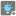• # Check fails an input that assert passes

## Question related to missionMultiple Lightbulbs

So, the following input:

```[
new Date(2015, 1, 12, 10, 0, 0),
[new Date(2015, 1, 12, 10, 0, 0), 2],
new Date(2015, 1, 12, 10, 0, 10),
[new Date(2015, 1, 12, 10, 1, 0), 2],
]
```

My code passes it normally in the asserts (it's the very first assert), but when checking it fails on Test 30, which is this same input. I can't figure out why. When I run the code with this input on my PC it gives the right answer too. My code:

```function sortFunc(a,b) {

var A
var B

if (a instanceof Array) {A = a}
else {A = a}

if (b instanceof Array) {B = b}
else {B = b}

return A > B ? 1 : -1
}

function sumLight(els, startWatching?, endWatching?) {

var bulbs = {1:0, 2:0, 3:0}
var out = 0
var bulb = 0

els.sort(sortFunc)

for (let el of els) {

if (el instanceof Array) {
var date = el
bulb = el
} else {
var date = el
bulb = 1
}

if (!(bulbs || bulbs || bulbs)) {
var start_time = date
}

bulbs[bulb] = 1 - bulbs[bulb]

if (!(bulbs || bulbs || bulbs)) {
var end_time = date

if (typeof startWatching != 'undefined') {
if (typeof endWatching != 'undefined') {
out += Math.max(0, Math.min(end_time - start_time, end_time - startWatching,
endWatching - start_time, endWatching - startWatching))
} else {
out += Math.max(0, Math.min(end_time - start_time, end_time - startWatching))
}
} else {
if (typeof endWatching != 'undefined') {
out += Math.max(0, Math.min(end_time - start_time, endWatching - start_time))
} else {
out += end_time - start_time
}
}
}
}

if (bulbs || bulbs || bulbs) {
if (typeof endWatching != 'undefined') {
if (typeof startWatching != 'undefined') {
out += Math.max(0, Math.min(endWatching - start_time, endWatching - startWatching))
} else {
out += Math.max(0, endWatching - start_time)
}
}
}
return out / 1000
}
```

Edit:

The checker claims that my function returns "null" for this input. So to test this, I replaced the return line by this:

```return out == null ? 60 : out / 1000
```

However, the checker still claims that my function returns "null". Seems to me that something is wrong with the checker.16